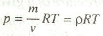# Characteristic Equation of a Gas

Characteristic Equation of a Gas is a modified form of general gas equation. If the volume (v) in the general gas equation is taken as that of 1 kg of gas (known as its specific volume, and denoted by vs), then the constant C (in the general gas equation) is represented by another constant R (in the characteristic equation of gas).

Thus, the general gas equation may be rewritten as:

p.vs= RT

where R is known as characteristic gas constant or simply gas constant.

For any mass m kg of a gas, the characteristic gas equation becomes :

m.pvs = mRT or pv = mRT

Notes:

1. In S.I. units, the pressure is expressed in bar (1 bar = 100 kN / m2).
2. The unit of gas constant (R), in S.I. units, is N-m / kg K or J / kg K (1 Nm = 1J)
3. The value of gas constant (R) is different for different gases. Its value for atmospheric air is taken 287 / kg K or 0.287 kJ / kg K.
4. The equation pv = mRT may also be expressed in another form i.e.,where ρ (rho) is the density of the given gas.